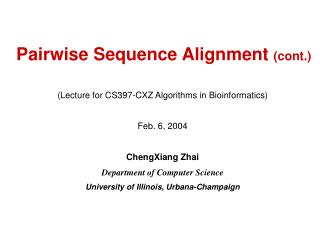Download PresentationPairwise Sequence Alignment (cont.)

# Pairwise Sequence Alignment (cont.) - PowerPoint PPT PresentationDownload Presentation## Pairwise Sequence Alignment (cont.)

- - - - - - - - - - - - - - - - - - - - - - - - - - - E N D - - - - - - - - - - - - - - - - - - - - - - - - - - -
##### Presentation Transcript

1. Pairwise Sequence Alignment (cont.) (Lecture for CS397-CXZ Algorithms in Bioinformatics) Feb. 6, 2004 ChengXiang Zhai Department of Computer Science University of Illinois, Urbana-Champaign

2. 4 Basic Questions in Pairwise Alignment (Modeling evolution) Q1: How should we define s? Q2: How should we define A? (Application-specific) Model: scoring function s: A X=x1,…,xn X=x1,…,xn Possible alignments of X and Y: A ={a1,…,ak} Find the best alignment(s) … S(a*)= 21 Y=y1,…,ym Y=y1,…,ym Q4: Is the alignment biologically Meaningful or just the best alignment of two unrelated sequences? Q3: How can we find a* quickly? (Dynamic programming) Q1 & Q4 are related! (Models for scores)

3. The Rest of This Lecture • Q4: How to assess the significance of an alignment score? • Classic approach: extreme value distribution • Bayesian approach: model comparison • Q1: How to define the scoring function? • Define the substitution score s • Define the gap penalty function g

4. First, Q4: Assessing Score Signficance • In general, larger s  more significant. The question is how large should s be? • Factors to be considered: • Sequence length: longer sequences are expected to give higher scores • # sequences in the database: the score of the best alignment is expected to be higher for a larger DB • Evolution time: longer evolution causes more mismatches, making a lower score more significant • The Challenge is how to quantify all these…

5. Two Basic Approaches • The classical approach: Extreme value distribution • Assume a null (random) model for scores M0 • P(Score > s|M0, x, y)=? • The Bayesian approach: Model comparison • Assume two models for (x,y): random M0; aligned: M1 • P(M1|x,y)/P(M0|x,y)=? prior Log-odds score of the alignment

6. Extreme Value Distribution • EVD: The asymptotic distribution of the maximum MN of a series of N independent normal random variables is • In general, the maximum of a large number of separate scores follows this distribution • Example: the best local match score between two long sequences constants mode

7. EVD of the Best Score in Ungapped Local Alignment • The number of unrelated local matches with score higher than S is approximately Poisson distributed, with mean • The probability that there is a match of score greater than S is • K and  can be fit using randomly generated data • This gives a way to test statistical significance p(x>21)= 0.01 vs. p(x>21)=0.3 Parameters Sequence lengths

8. Bayesian Model Comparison Assumptions: • M is a model for related sequences • R is a model for unrelated sequences (random) • Ungapped alignment n=m • Alignment of each pair is independent Score S(x,y) Prior (Subjective!) This partially addresses Q1: how to design the scoring function?

9. Q1: How to Estimate Probabilities? • General idea: Exploit sequences with known (“reliable”) alignments • Simplest method: Max. Likelihood estimator • Improved method: Consider evolution time (phylogenetic tree, to be covered later)

10. Dayhoff PAM Matrices • Estimate p(b|a,t,M) (Substitution probabilities) rather than p(ba|M) • Use sufficiently similar sequence pairs to estimate p(b|a,t=1,M) • Compute p(b|a, t+1,M) based on p(b|a,t,M) • Compute the score matrix (e.g., PAM 250)

11. BLOSUM Matrices • Limitation of PAM: short time substitutions are dominated by trivial changes in the Codon triplets • BLOSUM tries to improve the estimation of p(ab|M,t) by re-sampling the aligned, ungapped sequences regions (e.g., based on PAM) • Time t is now connected with a threshold of sequence similarity, leading to different variations (e.g., BLOSUM50 & BLOSUM62)

12. Estimating Gap Penalties • Again the basic idea is to exploit known alignments • Basic assumptions: • The gap-open score d is linear in log(t) • The gap-extend score e is constant • Example: (g)=A+B*log(t)+C*log(g) • In practice, people choose the gap costs empirically for given substitution scores.